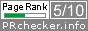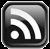# LEARN MS-ACCESS TIPS AND TRICKS

Learn Microsoft Access Advanced Programming Techniques, Tips and Tricks.

### Introduction.

Microsoft Access doesn't have this function built-in. I have made an attempt to write the code for this function and you may use it at your own risk. Before going to the code take a look at the usage examples of this function in Excel.

### ROUNDUP() function in Microsoft Excel.

#### The Rules.

Rounds a number up, away from 0 (zero).

Syntax: ROUNDUP(number,num_digits) Number is any real number that you want to be rounded up.

Num_digits is the number of digits to which you want to round number.

Remarks ROUNDUP behaves like ROUND, except that it always rounds a number up.

If num_digits is greater than 0 (zero), then the number is rounded up to the specified number of decimal places.

If num_digits is 0, then the number is rounded up to the nearest integer.

If num_digits is less than 0, then the number is rounded up to the left of the decimal point.

#### Examples:

=ROUNDUP(3.2,0) Rounds 3.2 up to zero decimal places (4)

=ROUNDUP(76.9,0) Rounds 76.9 up to zero decimal places (77)

=ROUNDUP(3.14159, 3) Rounds 3.14159 up to three decimal places (3.142)

=ROUNDUP(-3.14159, 1) Rounds -3.14159 up to one decimal place (-3.2)

=ROUNDUP(31415.92654, -2) Rounds 31415.92654 up to 2 decimal places to the left of the decimal (31500)

Courtesy: Microsoft Excel Help Documents.

Copy and Paste the following VBA Code into a Standard Module of your Database and try it out. The examples given above have been successfully passed on my testing of the code.

### The ROUNDUP() Function.

```Public Function ROUNDUP(ByVal dblNum As Double, ByVal intprecision As Integer) As Double
'-------------------------------------------------
'ROUNDUP() Function of Excel Redefined in MS-Access
'Author: apr pillai
'Date  : June 2016
'-------------------------------------------------
On Error GoTo ROUNDUP_Err
Dim sign As Integer
sign = Sgn(dblNum)
dblNum = Abs(dblNum)
dblNum = dblNum * 10 ^ intprecision
ROUNDUP = (Int(dblNum + (1 - IIf((dblNum - Int(dblNum)) <> 0, (dblNum - Int(dblNum)), 1))) / 10 ^ intprecision) * sign

ROUNDUP_Exit:
Exit Function

ROUNDUP_Err:
MsgBox Err & ": " & Err.Description, , "ROUNDUP()"
Resume ROUNDUP_Exit
End Function```

suggestions for improvement of the above VBA Code are welcome.

Share:

Comments subject to moderation before publishing.

## TranslateSubscribe in a readerYour email address:

Delivered by FeedBurner